• 深入浅出HALCON几何变换 在机器视觉系统中，镜头是重要成像部件之一，而基于小孔成像原理的工业镜头往往会产生透视畸变现象，如何校正畸变是进行图像分析的前提，这其中就会用到投影变换，也是几何变换的一种。除...

深入浅出HALCON几何变换

在机器视觉系统中，镜头是重要成像部件之一，而基于小孔成像原理的工业镜头往往会产生透视畸变现象，如何校正畸变是进行图像分析的前提 ，这其中就会用到投影变换，也是几何变换的一种。除此之外，图像处理中常用到的平移、旋转、缩放等，也属于几何变换。本文将通过实例详细介绍HALCON中的几何变换。

基础知识

齐次坐标（Homogenous Coordinate）

齐次坐标就是将一个原本是n维的向量用一个n+1维向量来表示。例如二维点p(x,y)àp(x,y, w)就成了齐次坐标，同理三维点p(x,y,z)àp(x,y,z, w)也成了齐次坐标。显然，齐次坐标的表达并不是唯一的，随w值的不同而不同。在计算机图学中，w 作为通用比例因子，它可取任意正值，但一般在几何变换中，总是取w=1。齐次坐标表示是计算机图形学的重要手段之一，它既能够用来明确区分向量和点，同时也更易于进行几何变换。

以点p(x,y)为例，如果想把它平移至p(x+a,y+b)，是不可能用矩阵计算完成的，现在换成齐次坐标(x,y,1),通过矩阵相乘(左侧公式) ，很方便得到平移后的坐标(x+a,y+b)。通常把变换矩阵写到左侧，为了保持一致把变换矩阵改成右下角形式，这就是齐次变换矩阵。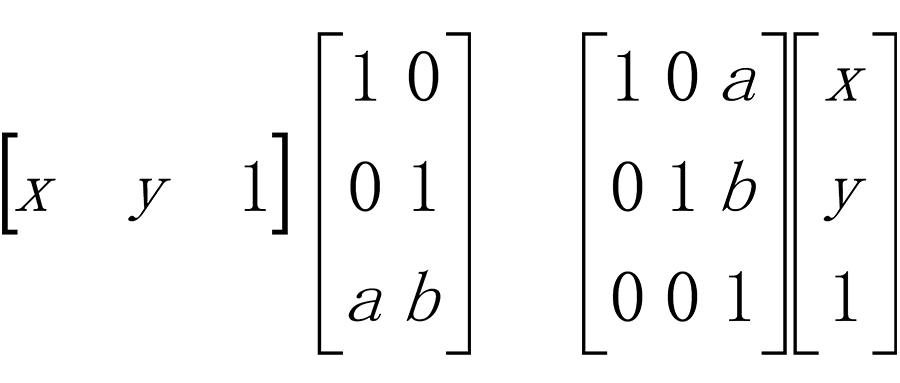齐次变换矩阵

在同一变换矩阵中既表示姿态（旋转、缩放等）又表示位置（平移），这种形式的矩阵称为齐次变换矩阵。如下：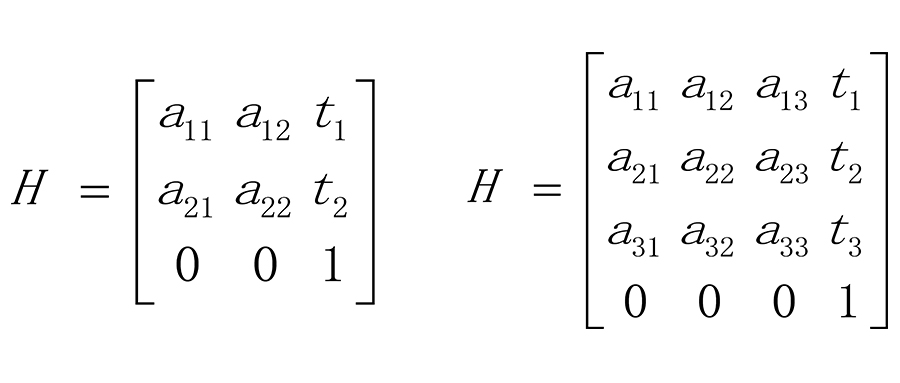齐次坐标的使用，使得几何变换更容易计算，尤其对于仿射变换（二维/三维）更加方便，由于图形硬件、视觉算法已经普遍支持齐次坐标与矩阵乘法，因此更加促进了齐次坐标使用，使得它成为图形学中的一个标准，下面提到的几何变换（仿射变换）都以齐次坐标和齐次变换矩阵为基础。

几何变换

几何变换包括：

相似变换（Similarity Transformation）

仿射变换（Affine Transformation）

投影变换（Projective Transformation）

注：线性变换不包含平移，所以没有归入几何变换，总之HALCON中常用几何变换是仿射和投影变换，而相似变换是仿射变换的一种简单情况！相似变换、仿射变换、投影变换既可以发生在二维空间内也可发生在三维空间内。

相似变换

相似变换的定义：由一个平面/立体图形变换到另一个平面/立体图形，在改变的过程中保持形状不变（大小方向和位置可变），任何相似变换都可以分解为等比例缩放、平移、旋转的组合，例如，对于缩放，用齐次变换矩阵可以如下表示(二维和三维），其中a≠0。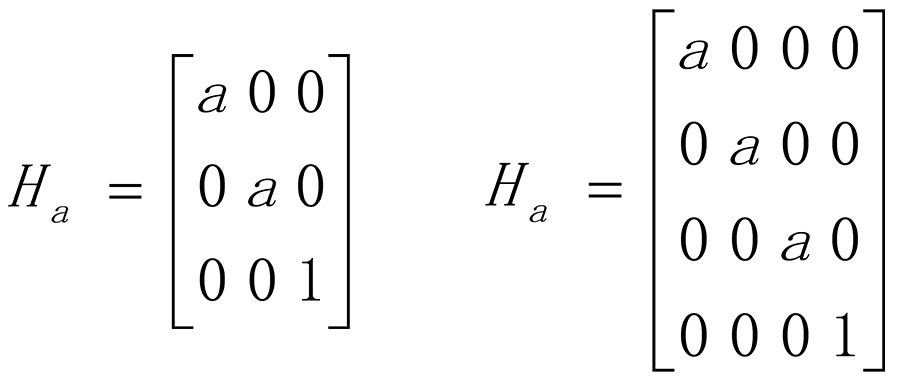仿射变换

仿射变换的定义：由一个平面/立体图形变换到另一个平面/立体图形，在改变的过程中保持直线和平行线不变(平行线映射为平行线），任何仿射变换都可以分解为缩放、平移、旋转和切变(Shearing)的组合，对于仿射变换，齐次变换矩阵如下表示(二维和三维）：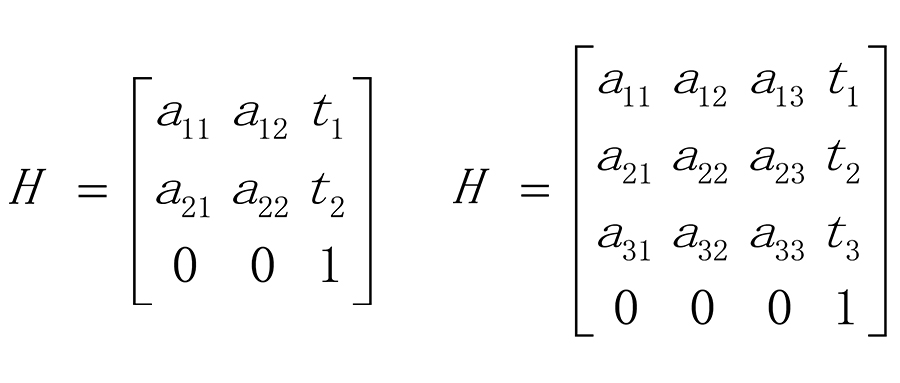对于仿射变换，有两个比较特殊的变换：非等比例缩放和切变（如下图），除了这两个特殊的变换之外，相似变换可以看做是仿射变换的特殊形式，注：线性变换包括旋转、缩放、切变，但不包含平移，因此仿射变换也定义为一个线性变换再加上一个平移变换。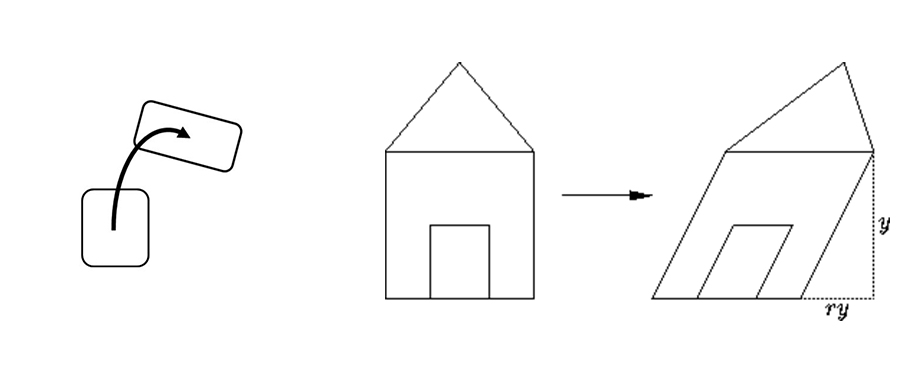投影变换

投影变换的定义：变换过程中，直线映射为直线(但不一定保证平行度)，任何二维投影变换都可以用3x3可逆矩阵表示，而任何三维投影变换都可以用4x4可逆矩阵表示。如下：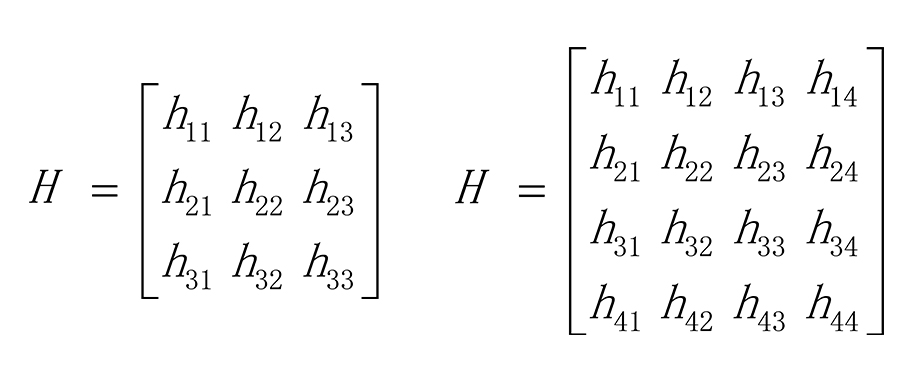从定义来看，仿射变换可以看做是投影变换的特殊形式。另外，把投影变换矩阵的最后一行变为[0,0,1]或者[0,0,0,1]，即可变为仿射变换矩阵，也可以证明仿射变换是投影变换的特殊形式。因此，对于平移、缩放、切变等，仿射变换和投影变换都可以实现。

如下例子，把左图进行旋转操作变成右图，可以用仿射变换和投影变换两种方式实现。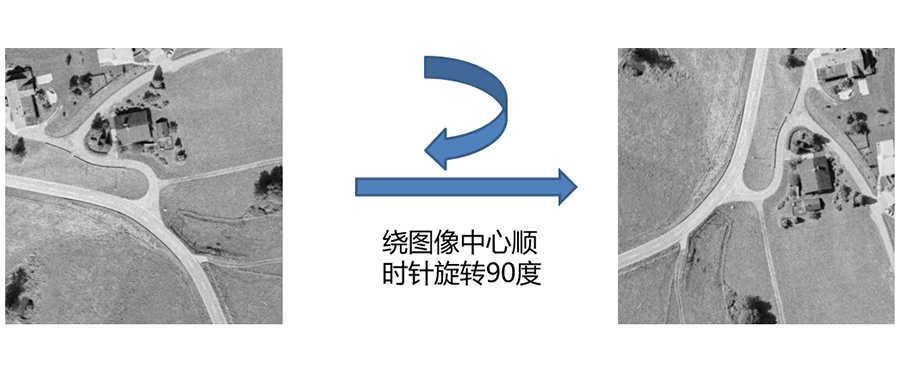仿射变换实现方法

hom_mat2d_identity (HomMat2DIdentity)

hom_mat2d_rotate (HomMat2DIdentity, rad(-90), 256, 256, HomMat2DRotate)

affine_trans_image (Image, ImageAffinTrans, HomMat2DRotate, 'constant', 'false')

投影变换实现方法之一
（直接用给定点生成投影变换矩阵）

hom_vector_to_proj_hom_mat2d([0,0,512,512],[0,512,512,0],[1,1,1,1], [512,512,0,0], [1,1,1,1], ‘dlt', HomMat2D)

projective_trans_image (Image, TransImage, HomMat2D, 'bilinear', 'false', 'false')

投影变换实现方法之二
（用三维仿射变换矩阵生成投影变换矩阵）

注：对于旋转来说，也可以看做是相机沿Z轴旋转90度后的成像效果，因此也可以用三维仿射变换，先转换相机坐标系，再生成投影变换矩阵！

hom_mat3d_identity (HomMat3DIdentity)

hom_mat3d_rotate (HomMat3DIdentity, rad(90), 'z', 256, 256, 512, HomMat3DRotate)

hom_mat3d_project (HomMat3DRotate, 256, 256, 512, HomMat2D)

projective_trans_image (Image, TransImage, HomMat2D, 'bilinear', 'false', 'false')

旋转示例中的仿射变换矩阵（也是齐次变换矩阵）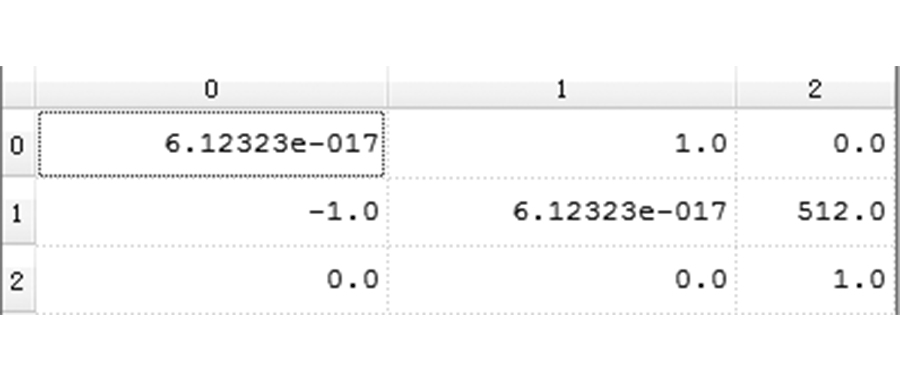旋转示例中的投影变换矩阵（3X3），也是可逆矩阵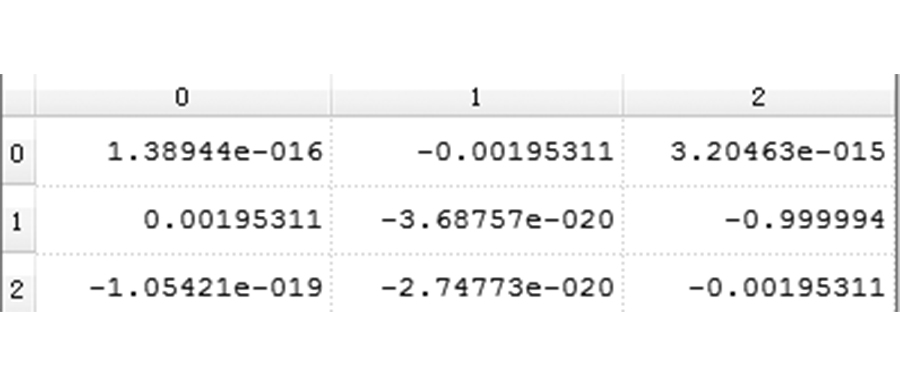同理，缩放、切变等，仿射变换和投影变换也都可以实现，但如下变换，只能用二维投影变换矩阵实现，因为变换过程中没有保证线的平行性。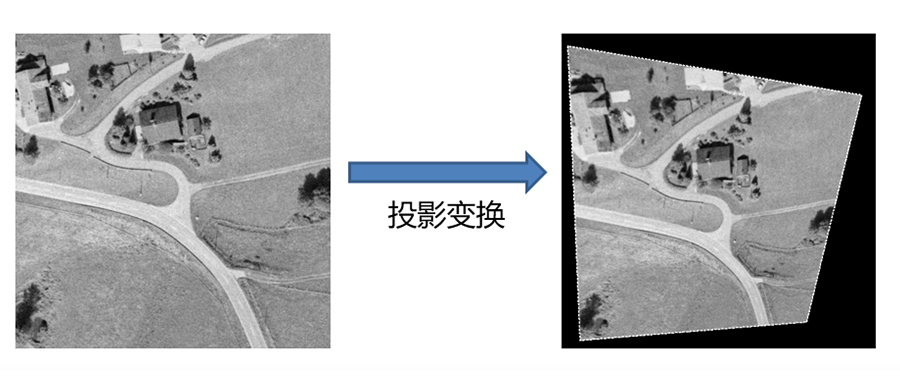S

总结

HALCON中所用的变换知识只涉及到了二维、三维仿射变换和二维投影变换，二维仿射变换多用于平面图像的平移、旋转、缩放等；三维仿射变换多用于三维坐标系的平移、旋转等（或者用3D位姿表示），而二维投影变换多用于校正透视畸变，也就是三维空间的景象如何投影到相机靶面上的效果，如果相机和被测平面不垂直就会有畸变。

在成像过程中，普通工业镜头（小孔成像原理）都会带来透视畸变，也就是常见的近大远小现象，除非相机和被测平面保持绝对垂直，否则透视畸变是不可避免的。因此，通过三维空间的仿射变换（变换坐标系，使相机不垂直于被摄平面），可以产生透视畸变效果，也就是相当于进行了投影变换，HALCON也提供了算子hom_mat3d_project，能直接把三维仿射变换矩阵转换成二维投影变换矩阵（4x4的矩阵转换成3x3矩阵）。下面我们通过一个例子来说明一下。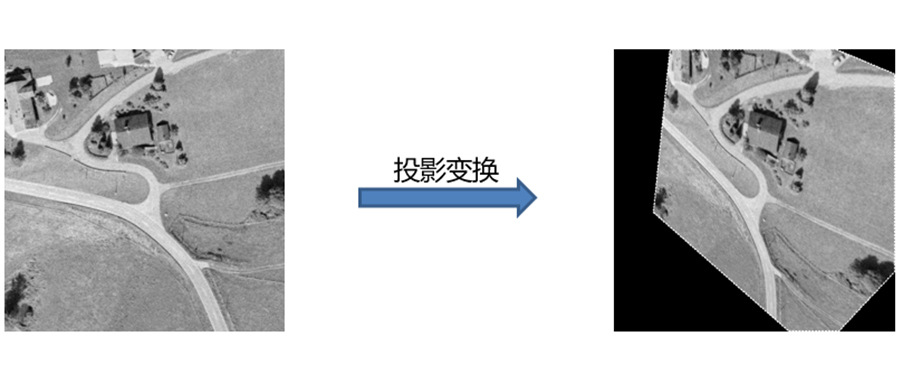首先生成单位矩阵，进行一系列的旋转变换，产生三维仿射变换矩阵，也就是使得相机和被摄平面不垂于！

hom_mat3d_identity (HomMat3D)

hom_mat3d_rotate (HomMat3D, rad(20.0), 'z', PrincipalRow, PrincipalColumn, Focus, HomMat3D)

hom_mat3d_rotate (HomMat3D, rad(35.0), 'y', PrincipalRow, PrincipalColumn, Focus, HomMat3D)

hom_mat3d_rotate (HomMat3D, rad(25.0), 'x', PrincipalRow, PrincipalColumn, Focus, HomMat3D)

把三维仿射变换矩阵再转化成二维投影变换矩阵

hom_mat3d_project (HomMat3D, PrincipalRow, PrincipalColumn, Focus, ProjectionMatrix)

进行投影变换，也就是我们看到的右侧的效果

projective_trans_image (Image, TransImage, ProjectionMatrix, 'bilinear', 'false', 'false')

另外一种方法是，直接使用给定点生成投影变换矩阵，可以得到同样的效果

hom_vector_to_proj_hom_mat2d ([0,0,512,512], [0,512,512,0], [1,1,1,1], [-120,146,555,296], [120,752,368,72], [1,1,1,1], 'normalized_dlt', HomMat2D)

projective_trans_image (Image, TransImage1, HomMat2D, 'bilinear', 'false', 'false')

上面的这个例子是很好的说明了透视畸变现象如何产生的，左侧图是相机垂直于被摄平面，没有透视变形现象。然后进行了一系列的三维仿射变换，产生的效果就是相机不再垂直于被摄面，所以右图产生了透视畸变现象，那么从左图到右图恰恰是进行了一次二维投影变换。因为投影变换矩阵是可逆的，所以也可以把右图校正为左图，这恰恰是HALCON中投影变换的用处所在！

以下例子也是HALCON中典型的畸变校正示例，使用的正是投影变换。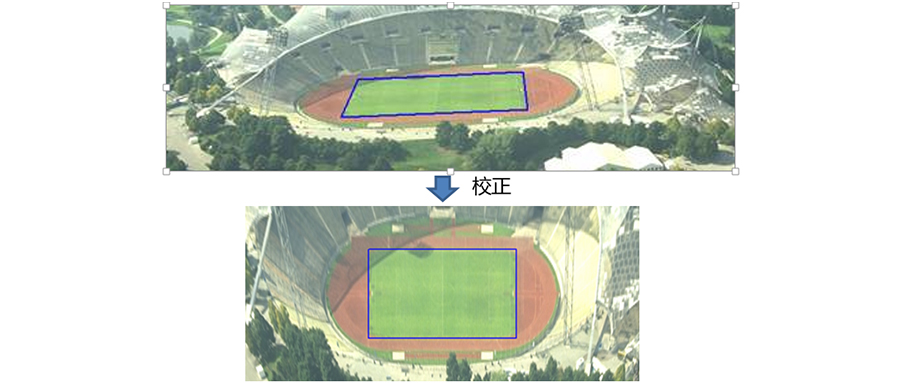附录

解释一下HALCON中的：

hom_mat2d_*和hom_mat3d_*算子

hom_mat2d_identity产生2x3矩阵，用于描述二维变换，实际上是3x3齐次变换矩阵(仿射变换)；

hom_mat3d_identity产生3x4矩阵，用于描述三维变换，实际上是4x4齐次变换矩阵(仿射变换)；

hom_mat2d_*既支持2x3矩阵（仿射变换）也支持3x3矩阵（投影变换）;

hom_mat3d_*支持3x4矩阵（仿射变换），也就是4x4齐次变换矩阵

关于如何在HALCON中生成的：

仿射变换矩阵和投影变换矩阵

仿射变换矩阵

用hom_mat2d_identity或者hom_mat3d_identity产生单位矩阵，然后经过平移、旋转、缩放等操作后得到仿射变换矩阵。

投影变换矩阵

hom_mat3d_project能直接把三维仿射变换矩阵转换成投影变换矩阵，如果知道空间坐标系是如何变换的，可以用这种方法；

hom_vector_to_proj_hom_mat2d用给定点生成投影变换矩阵，输入点的坐标为三维齐次坐标，可以支持无穷远点和有限远点的变换；

vector_to_proj_hom_mat2d用给定点生成投影变换矩阵，输入点为二维非齐次坐标，支持有限远的点的变换；

注：对于涉及有限远点的变换，以上两个算子都可以！


展开全文• 几何变换非常常见，在计算机视觉和图形学上更是如此，而这里指的几何一般是由点，线，面等几何元素组成的1，2维或3维图形。几何变换能够实现不同空间几何元素的对应，在很多领域中有着非常多的应用，立体视觉便是...

【多视角立体视觉系列】 几何变换的层次——投影变换，仿射变换，度量变换和欧几里德变换

20200226 FesianXu

文章目录前言你不可不知的几何元素直线点面线的相交圆锥线和二次曲锥面说在前面——理想点和无限远处的线和面走得更进一步——讨论几何变换投影几何空间和齐次坐标系更配哦~数学形式的四大类型几何变换投影变换仿射变换相似性变换欧几里德变换总结Reference

前言
几何变换非常常见，在计算机视觉和图形学上更是如此，而这里指的几何一般是由点，线，面等几何元素组成的1，2维或3维图形。几何变换能够实现不同空间几何元素的对应，在很多领域中有着非常多的应用，立体视觉便是其中一个。本文尝试对四种不同类型的几何变换进行辨析，这些几何变换是一系列计算机视觉处理和相机成像的基础，因此有必要进行掌握。如有谬误，请联系指出，转载请注明出处。
$\nabla$ 联系方式：
e-mail: FesianXu@gmail.com
QQ: 973926198
github: https://github.com/FesianXu
知乎专栏: 计算机视觉/计算机图形理论与应用
微信公众号：你不可不知的几何元素
研究一个几何问题，一般可以通过两种方式进行，第一种是进行“纯粹”的几何研究，也就是说这种研究应该是和所谓坐标系一点关系都没有的，在不使用任何代数方法的情况下，用几何公理推出其他定理，也就是传统的欧几里德几何；第二种，我们其实也是很熟悉的，就是解析几何，由笛卡尔提出并流行，在这种方法中，我们用代数元素，比如向量去表示几何的点，线，面，用点乘，叉乘去表示元素的一系列操作，从而可以用代数方法进行几何关系的推理。由于现在计算机本质上是对数值进行计算，因此通过解析几何的方法，也就是代数法更容易设计计算机可以解决的算法，因此本文首先要对几何元素进行代数表示。
正如我们在前言所说的，几何元素无非就是点线面，我们在中其实已经初步探讨过为什么要引进齐次坐标这个概念，然而，这里希望重新进行解释，因此我们将从线的代数表示开始说起。
直线
我们由中学的知识，知道线在二维空间可以表示为：
\begin{aligned} ax+by+c = 0 \end{aligned} \tag{1.1}
这个线完全由$l_1 = (a,b,c)^\mathrm{T}$ 的组合给决定了，因此可以用$l_1$这个向量表示这个直线，我们又注意到对于该线来说，式子(1.2)同样成立：
$(ka)x+(kb)y+(kc) = 0 \tag{1.2}$
而显然，$l_2 = k(a,b,c)^\mathrm{T}$和$l_1$表示的是同一个直线，于是我们知道，对于这条直线，其表示有无穷多种，此尺度大小也就是$k$只要不为0都是等价的。 特别的，如果$k = \dfrac{1}{c}$那么我们的原先的$c$就变为了1，于是该直线变成了$l_1 = (a,b,1)^\mathrm{T}$，其实只需要两个变量就可以决定一个2D直线了。
需要注意的是，本文用到的每个几何元素都是以列向量的形式表示的，也就是说$l_1 = (a,b,c)^\mathrm{T}$是一个列向量，而其转置$(a,b,c)$ 才是行向量。
点
怎么表示一个在线上的点呢？既然点在线上，那么自然地，满足式子(1.1)，如果把这个式子写成向量乘法的形式，我们有：
$(x,y,1)(a,b,c)^{\mathrm{T}} = (x,y,1)l = 0 \tag{1.3}$
说明点$p = (x,y,1)^\mathrm{T}$在该线上。为什么一个二维的点，会需要在尾巴上多一个1去表示呢？显然，这个是我们之前提到的齐次坐标系，但是为什么要这个坐标系，我们虽然之前也提到过，但是本文后面还会继续解释的。
面
面，一般是存在于三维几何中的概念，其可以表达为：
\begin{aligned} ax+by+cz+d &= 0 \\ (x,y,z,1)(a,b,c,d)^{\mathrm{T}} &= 0 \\ (x,y,z,1)P &= 0 \end{aligned} \tag{1.4}
也就是说，面可以用$P = (a,b,c,d)^\mathrm{T}$表示，而在面上的点，同样的是在齐次坐标下表示的，为$p = (x,y,z,1)^\mathrm{T}$。
我们发现到，无论是点线面，都可以通过一个向量进行表示。
线的相交
考虑在二维情况下，我们要如何表示两个线的交点呢？假如给定两个直线$l_1 = (a,b,c)^\mathrm{T}$, $l_2 = (a^{\prime}, b^{\prime}, c^{\prime})^\mathrm{T}$ ，定义出向量$x = l_1 \times l_2$，其中的$\times$表示的是叉乘。由向量叉乘的几何意义我们知道，其得到的是正交于两个向量的向量，显然，这两个向量中的任意一个向量正交于$l$，也就是有$l_1 \cdot (l_1 \times l_2) = 0, l_2 \cdot (l_1 \times l_2) = 0$ 也就是$l_1^{\mathrm{T}}x=l_2^{\mathrm{T}}x=0$ ，也就是说向量$x$同时通过了这两条线，显然，他就是交点。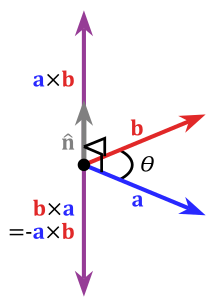Fig 1.1 向量的叉乘，其结果向量的方向正交于其中的任意一个向量。

于是我们知道，两个线的交点就是两个线表示的向量的叉乘：
$x = l_1 \times l_2 = [l_1]_{\times} l_2 \tag{1.5}$
其中的$l_1 = (a,b,c)$，而$[l_1]_{\times}$是一个矩阵，为：
$[l_1]_{\times} = \left[ \begin{matrix} 0 & -c & b \\ c & 0 & -a \\ -b & a & 0 \end{matrix} \right] \tag{1.6}$
圆锥线和二次曲锥面
圆锥线和二次曲锥面在计算机视觉的几何变换中特别常见，鉴于其篇幅较长，我独立成一篇博文，见。当然，你也可以先不管这个几何元素，直到我们后面提到了IAC, Image of Absolute Conic的时候在回过头复习它。

说在前面——理想点和无限远处的线和面
我们在中曾经讨论过理想点（Ideal point）这个概念，简单来说就是平行线交于无穷远处，这个定义可能不够直观，我们借助解析几何，用代数的形式，在齐次坐标的帮助下去定义它。考虑到两个平行线$l_1: ax+by+c_1 = 0, l_2:ax+by+c_2=0$，那么可以表示为$l_1 = (a,b,c_1)^{\mathrm{T}}, l_2 = (a,b,c_2)^{\mathrm{T}}$，那么由我们上面讨论的，我们知道两个平行线的交点，即便它们是平行线，也可以用叉乘去描述，如$l_1 \times l_2 = (c_2-c_1)(b,-a,0)^{\mathrm{T}}$，忽略这个尺度因子$(c_2-c_1)$，我们发现其交点是$(b,-a,0)^{\mathrm{T}}$。如果把这个齐次坐标转变为非齐次坐标，我们有$(b/0, -a/0)^{\mathrm{T}}$，这个显然是无法计算的，这也意味着平行线的交点都在无穷远处的点上，为了在欧几里德空间中表示这种无穷远处的点，非齐次坐标系是无能为力的，我们只能引入齐次坐标系，也就是在欧几里德空间坐标的基础上，在最后一维再加上一个维度。
（PS： 为什么非得在欧几里德空间里面，以统一的方式去表达平行线和非平行线的方程呢？那是因为，投影变换中，特别是透视变换中，存在将“平行线”变换成“非平行线”，或者将“非平行线”变换成“平行线”的操作，如果不能进行统一表示，那么就不能用统一的形式去数学形式化这个过程了。）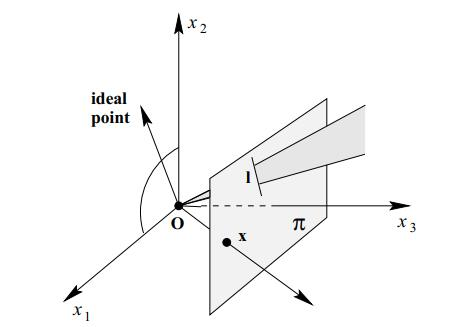Fig 2.1 即便是平行线也会在无限远处相交，这个相交点称之为理想点。

为了考虑平行线的相交的情况，对于二维平面，我们在无限远处假设出了无限远的线(line at infinity)，表示为$\mathbf{I}_{\infty}$ 。对于三维空间来说，我们在无限远处假设出了无限远的平面(plane at infinity)，表示为$\Pi_{\infty}$。我们尝试用代数的方式表示这两个元素，我们知道无限远处的点可以表示为$\mathbf{p}_{\infty} = (1,1,0)^{\mathrm{T}}$（三维情况下要多加一个维度），而无限远处的点应该在无限远处的平面或者线上，那么有
$\mathbf{I}_{\infty}^{\mathrm{T}} \mathbf{p}_{\infty} = 0 , 二维情况 \\ \mathbf{\Pi}_{\infty}^{\mathrm{T}} \mathbf{p}_{\infty} = 0 , 三维情况 \tag{2.1}$
这样就不难得到，这两个元素的一种表达方式为
$\mathbf{I}_{\infty} = (0,0,1)^{\mathrm{T}} \\ \mathbf{\Pi}_{\infty} = (0,0,0,1)^{\mathrm{T}} \tag{2.2}$
事实上，根据齐次坐标的性质，我们容易知道这两个元素的任意表达方式都是等价的。

走得更进一步——讨论几何变换
在之前的章节中，我们用代数的方式定义了很多几何元素，这些几何元素都是在几何变换中的基本变换单元，在本章节，我们将正式起航，讨论在空间中几何变换。我们首先要考虑的是最为熟悉的欧几里德空间，我们日常生活一般可以建模为欧几里德空间，可以定义出一个原点，然后两个或者三个互为正交的坐标轴，然后客体，也就是我们要研究的物体主体就在这个欧式空间中移动，旋转等，我们会发现，这里如果把客体看成是一个刚体，也就是自身不发生形变的物体，那么客体在欧式空间的旋转，平移等，都是所谓的欧几里德变换(Euclidean transformations)。当然这章暂时只是概念上的辨析，就先不拿出变换公式搞晕各位读者吧。
好的我们继续，注意到，虽然欧几里德空间坐标系一般都有一个原点，有相应的坐标轴，但是这个原点并没有什么特别的地方，坐标轴的方向也没有任何特别的地方，都是我们研究人员为了方便自己设定的，事实上，这个原点和坐标轴我们可以任意的指定，任何一个在有限空间内的，可以用代数表达的原点和坐标轴方向我们都可以指定，只要满足约束条件：

在有限的空间内的，也就是每个原点的分量值都是实数；
坐标轴互相正交

欧几里德空间里面的点都是 同质（homogeneous 的，意味着每个在欧式空间的点都是等价的，因此你在平移原点坐标，旋转坐标轴的同时，其实也是在进行着一系列的欧式变换。显然了，欧式变换并不能改变客体的实际长度，毕竟是看作刚体而研究的，同时也改变不了客体的线与线之间的角度，当然，平行线更是不会被改变了，原来相对平行的线，经过欧式变换后仍然还是相对平行的，如Fig 3.1所示。这个当然不是理所当然的，几何变换很多是不保留这些几何元素的，如果大家学过绘画或者摄影，就会发现所谓的透视原理就是典型的一种，不过这个暂且作为后话吧。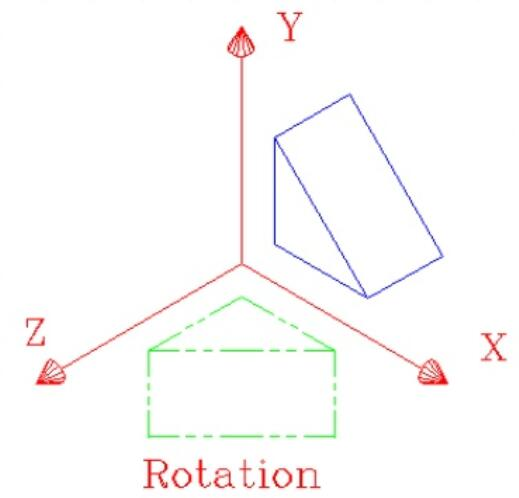Fig 3.1 欧式变换之一的旋转，我们发现，原来是直角的，变换后还是直角，原先平行的直线，转换后还是直角。

我们对欧几里德变换有了初步的认识，那么欧几里德变换是不是在研究工作中就足够使用了呢？很遗憾，显然不是的，比如计算机图形学中那么多需要对图形进行放大，缩小的操作在欧几里德变换中显然是失效的。因此我们还需要定义一种变换，能使得放大，缩小能够操作起来有理论依据。
这个其实并不困难，我们只需要在保持原点位置，坐标轴的指向方向不变的情况下，将每个坐标轴都“拉伸”或者“缩小”相同的倍数就行了，注意，是每个坐标轴都是相同的倍数。如果结合起欧几里德变换，那么我们就会发现我们可以旋转，平移，放大缩小我们的研究客体了，我们将其称之为相似性变换(similarity transformations)或者度量变换(metric transformations)。注意到，相似性变换包括了欧几里德变换，即是 $G\{欧几里德变换\} \subseteq G\{相似性变换\}$。
然而，有了放大缩小，我们似乎还是还缺少了一些变换工具，去描述客体在某个特定方向的单独的拉伸或者缩小，而这在某些特殊情况下的成像中是必须的工具（见[5,6]中的仿射相机部分知识点）。于是我们引入了仿射变换(affine transformation)，在仿射变换中，每个轴不再是像相似性变换中一样都是放缩同样的倍数了，而是可能放缩不同的尺度。注意到，仿射变换包括了相似性变换和欧几里德变换，即是 $G\{欧几里德变换\} \subseteq G\{相似性变换\} \subseteq G\{仿射变换\}$。
这样足够了吗？我们成像出来的物体，在引入了仿射变换之后，也就是如果我们用仿射相机去拍摄一个正方体，会形成如Fig 3.2所示的效果的平面图形（当然，忽视虚线部分）。我们会发现，如果光从这个平面图形，我们完全没法推断出这个客体在三维立体空间的深度信息，客体因为在空间中各个部分距离相机中心的距离或多或少有所不同，正如我们在中的“透视投影的若干性质”中曾经讨论过的，这种因为客体深度不同本应该导致投影缩放（foreshortening）在内的投影变形，而这种变形 表现出来就是远小近大，在客体某些线条就算本身尺度上是一样的，在投影的平面上都可能会产生一定的比例关系。 这种变形有时候正是我们想要的，对于我们人类从平面图形中理解客体在立体工具的深度是不可或缺的存在。因此，如果光用仿射相机，那么形成出来的二维图像就完全失去了推断出客体深度的信息。（如果你的素描老师看到你画的图如Fig 3.2所示，大概会直接挂科吧，RIP）。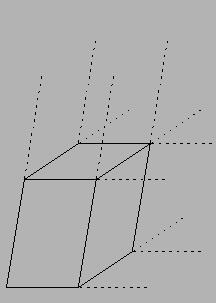Fig 3.2 在仿射相机角度下的立方体，是没有任何立体感的，因为其线条长度的比例不能体现因为客体不同部件距离相机的距离不同而导致的投影变形，这种变形对于我们人类在平面上认识客体的深度信息，却是非常关键的。

我们该怎么办？相机的初衷是在平面上对客体，对大自然进行复刻，那么自然想要保存更多的原始信息，丢失了深度信息可完全不划算，因此我们还需要引入一个变换形式，我们称之为投影变换（projective transformations），注意到，投影变换包括了仿射变换，相似性变换，欧几里德变换，也就是
$G\{欧几里德变换\} \subseteq G\{相似性变换\} \subseteq G\{仿射变换\} \subseteq G\{投影变换\}$
因此投影变换是一个非常大的种类，其中可以解决我们刚才提到的，在二维平面上体现三维客体的深度信息的成像方法，称之为透视法（perspective），学素描和摄影的读者应该对这个术语很熟悉吧。透视法的原则就是远处的物体看起来小，近处的物体看起来大，所谓的“远小近大”，在透视法中，平行线是会在无穷远处相交的，这个相交点可以称之为消失点（vanish point），联想到我们曾经定义的理想点的概念，我们知道消失点便是理想点。透视法呈现的图像如Fig 3.3所示：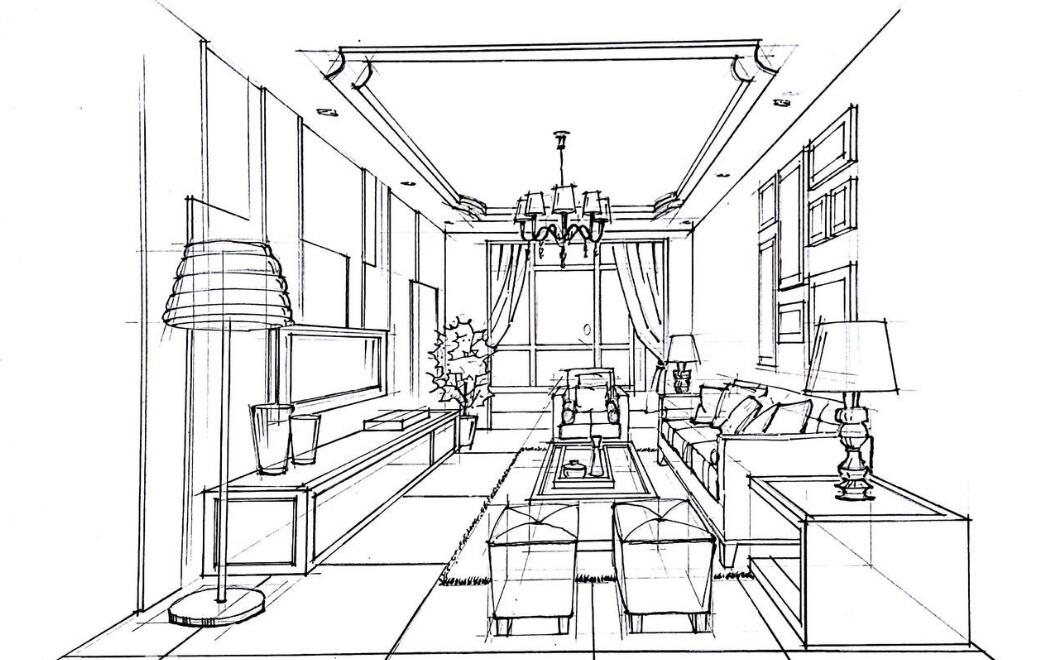Fig 3.3 通过透视法绘画得到的场景，可以通过计算线条之间的相对比例从而恢复出相对景深的大小，当然绝对景深光从比例还是不够的，例如你不可能知道这个椅子的绝对尺寸是多少，但是你可以推算出每个椅子之间的具体尺寸比例。

从Fig 3.3中，我们可以通过计算线条比例，从而对场景客体的相对景深进行一定程度上的重建，当然这个重建不是完美的，我们需要很多后续讨论的工具才能更好地进行重建。但是，起码通过投影变换中的透视法，我们能够在二维图像上保存更多的三维客体的信息了。
这里我要插个嘴，到底什么叫做投影（projectivity）呢？我们最为直观的印象就是太阳光照着不可透光的房子，形成的倒影，如Fig 3.4所示。这个直观感觉是正确的，确实我们也是这样定义的：

投影性（projectivity）是一种映射$h$，其可以从投影几何空间$\mathbb{P}^{2}$（当然也可以是三维的，见下一章节的介绍）映射回这个投影几何空间，使得当且仅当$h(\mathbf{x_1}),h(\mathbf{x_2}),h(\mathbf{x_3})$三点共线时，$\mathbf{x_1},\mathbf{x_2},\mathbf{x_3}$也是共线的。

因此投影线也称之为单应性（homography）或者共线性（colineation），显然共线性这个名称更为形象生动。投影性其实表示的是在投影前后，直线还是直线，该共线的点还是共线的，就那么简单，完事儿。不过多说一句，这种共线性可以用矩阵形式表达，如在二维空间中(用的是齐次坐标系)：
$h(\mathbf{x}) = \mathcal{H} \mathbf{x}, \mathcal{H} \in \mathbb{R}^{3 \times 3} \tag{3.1}$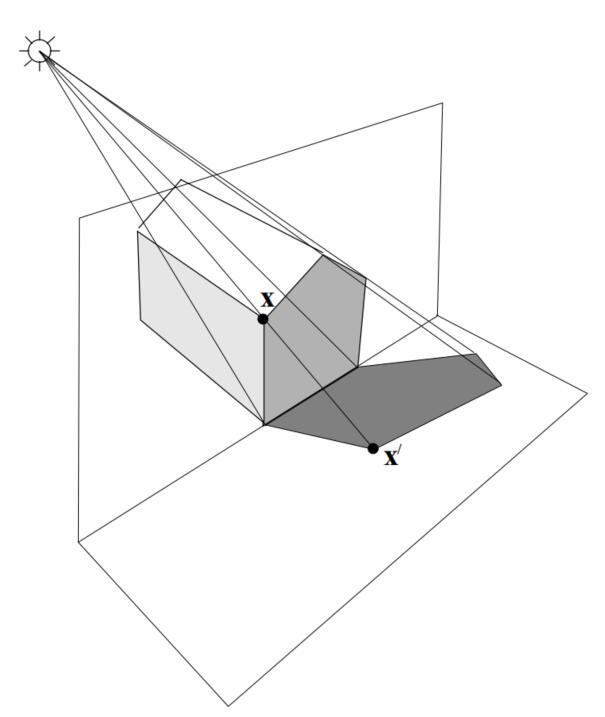Fig 3.4 投影，我们的直观印象就是太阳光在不可透光客体上的形成的影子。

这就是我们暂时的所有变换了：欧几里德变换，相似性变换，仿射变换和投影变换。当然，在本章节只是从感性的角度去理解这些变换的概念，我们接下来才是正式地步入深入理解这些变换背后的数学含义的章节，让我们继续吧。

投影几何空间和齐次坐标系更配哦~
是的你没看错，这一章又会和齐次坐标系扯上关系了，不过我们还是从欧几里德几何空间说起吧。还记得我们的欧几里德空间吗，在这个空间里面的变换不管你怎么变换，无限远处的点永远都在无限远处，而有限的点永远都不可能跑到无限远处对吧，这个是显而易见的。这个性质同样在相似几何空间，仿射几何空间成立，有限远的就是有限远的，无限远的就是无限远的，各自为政，谁也不干扰谁。因此在这些空间去描述变换，实际上并不需要齐次坐标系，只需要非齐次坐标系就足够了，因为我们根本就不需要去描述理想点。
但是，我们还有个投影几何空间，而在这个空间里面，几何变换是很“任性”的，变换前后平行性是得不到保证的，为什么呢？因为正是没办法保证平行性，我们才能提供视觉上的深度信息，这个正是我们想要的。平行性得不到保证，意味着变换前是平行的线，我们知道其交点在理想点处，变化后就可能不再平行了，那么其交点就变成了在有限远处的一个点了，反过来也是成立的。这个在非齐次坐标系下根本没办法解决，毕竟非齐次坐标连理想点和理想线，理想面都没办法描述，又怎么能描述其变换过程呢？因此我们正式引入齐次坐标系，在投影几何空间中，我们必须使用齐次坐标系描述变换过程，为了四个种类变换的公式表达的形式上的统一，我们对于这四种几何空间的变换，一致性地采用齐次坐标系。
那么假设欧几里德空间用$\mathbb{R}^{2},\mathbb{R}^3$表示，为了体现投影几何空间的特殊性，我们干脆给他一个表示吧，就表示为$\mathbb{P}^2, \mathbb{P}^3$，顺便我们也给仿射空间一个表示$\mathbb{A}^{2}, \mathbb{A}^3$ 。（别伤心啦，你其实不是顺便的，后面我们还用得上呢，嘿嘿）。

数学形式的四大类型几何变换
在用数学形式描述四大类型的几何变换之前，我们要先探讨下，到底什么叫“几何变换”？几何变换不应该只是几个公式咻咻咻地套进去，然后从一堆数字到另一堆数字的过程，几何变换的过程中，我们要留意的是，到底什么几何元素一直没有改变，而什么几何元素可能会改变的。这种变换前后的不变性，对于研究几何变换来说是很重要的，变换的不变性在计算机视觉中也会提供很重要的点子，是一个不可忽视的要点。不管怎么说，我们接下来要留意几何变换的不变性了。注意，接下来的讨论都在三维空间的例子中讨论，涉及到二维空间时将会特别提醒。
投影变换
因为投影变换的范围是最广的，其数学形式是最为通用的，于是我们就先从投影变换开始讨论吧。正如式子(3.1)所展示的，我们可以通过线性矩阵变换来描述投影变换，如：
\begin{aligned} \mathbf{T}_{P} &= \left[ \begin{matrix} p_{11} & p_{12} & p_{13} & p_{14} \\ p_{21} & p_{22} & p_{23} & p_{24} \\ p_{31} & p_{22} & p_{33} & p_{34} \\ p_{41} & p_{22} & p_{43} & p_{44} \end{matrix} \right] \in \mathbb{R}^{4 \times 4} \\ \left[ \begin{matrix} X^{\prime} \\ Y^{\prime} \\ Z^{\prime} \\ 1 \end{matrix} \right] &= \mathbf{T}_P \left[ \begin{matrix} X \\ Y \\ Z \\ 1 \end{matrix} \right] \end{aligned} \tag{5.1}
注意到$\mathbf{T}_P$其实自由度是15，虽然他有16个元素，具体原因见中的关于自由度的说明。在投影变换中，只有共线性，切线性和交叉比(cross-ratio)是不变的。共线性我们之前说过了，切线性指的是，在变换前某个直线是某个曲线的切线，那么变换后这个性质同样保留。投影变换不保留平行性，也不保留无限远处的理想点的位置，因此变换前后，无限可能变成有限，反之亦然。
至于交叉比，我们这样理解，假设客体上有四个点共线，分别是$M_1, M_2, M_3, M_4$，那么在选定了参考点之后，其他的共线的点可以被如下式子统一表示：
$M_i = M+\lambda_iM^{\prime} \tag{5.2}$
那么交叉比表示为：
$\{M_1, M_2:M_3,M_4\} = \dfrac{\lambda_1-\lambda_3}{\lambda_1-\lambda_4}:\dfrac{\lambda_2-\lambda_3}{\lambda_2-\lambda_4} \tag{5.3}$
这个交叉比的具体比例和参考点的选定无关，并且其在投影变换下保持不变。这个性质为我们通过平面的几何体线条比例去计算客体的相对深度信息提供了依据。
如图Fig 5.1所示，这个是一个特殊的投影变换——透视法后的一个正方体的二维透视图。我们发现平行线相交于理想点$V_z, V_y, V_x$。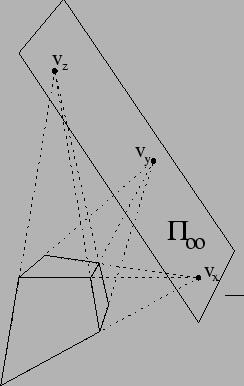Fig 5.1 透视法下的正方体二维图形。

具体的一些消失点的计算，可以参考我以前的博客，这里不赘述。
仿射变换
仿射变换的数学形式如(5.4)(5.5)所示，可以发现是对投影变换进行了一些元素上的约束后产生的，一般常见的仿射变换子类型包括旋转（rotation），尺度放缩（scale），平移（translation），切变（shear），具体的公式和约束条件见以前的博文。注意到仿射变换前后的不变性继承了投影变换的不变性，也即是共线性，交叉比和切线性。
\begin{aligned} \mathbf{T}_{A} &= \left[ \begin{matrix} a_{11} & a_{12} & a_{13} & a_{14} \\ a_{21} & a_{22} & a_{23} & a_{24} \\ a_{31} & a_{22} & a_{33} & a_{34} \\ 0 & 0 & 0 & 1 \end{matrix} \right] \in \mathbb{R}^{4 \times 4} \\ \left[ \begin{matrix} X^{\prime} \\ Y^{\prime} \\ Z^{\prime} \\ 1 \end{matrix} \right] &= \mathbf{T}_A \left[ \begin{matrix} X \\ Y \\ Z \\ 1 \end{matrix} \right] \end{aligned} \tag{5.4}
其$\mathbf{T}_A$的约束是：
$\det( \left[ \begin{matrix} a_{11} & a_{12} & a_{13}\\ a_{21} & a_{22} & a_{23}\\ a_{31} & a_{22} & a_{33} \end{matrix} \right] ) \neq 0 \tag{5.5}$
容易发现的是，仿射变换的自由度是12，并且容易可以验证仿射变换不会移动无限远处的理想面$\Pi_{\infty}$，可以简单证明下：
在仿射变换后，理想面变成
$\Pi_{\infty}^{\prime} = \mathbf{T}_A^{-\mathrm{T}} \Pi_{\infty} \tag{5.6}$
我们从(2.2)知道了对于理想面的表达为$\Pi_{\infty} = (0,0,0,1)^{\mathrm{T}}$。于是容易验证$\Pi_{\infty}^{\prime} = (0,0,0,1)^{\mathrm{T}}$因此未曾改变理想面。然而，在理想面上的理想点的位置即便没有从无限远处变成有限远处，在仿射变换下也可能在理想面上发生偏移，也就是说仿射变换不保留理想面上点的位置。这一点可以简单证明下，假设理想面上有一个圆锥线 $\mathbf{x}^{\mathrm{T}} \mathbf{C} \mathbf{x} = 0$其中$\mathbf{C} = \mathbf{I}_{3 \times 3}$单位矩阵，假设仿射变换后有点的位置偏移$\mathbf{x}^{\prime} = \mathbf{T}_A\mathbf{x}$，那么我们可以知道，变换后的$\mathbf{C}^{\prime} = \mathbf{T}_A^{\mathrm{T}} \mathbf{C} \mathbf{T}_A = \mathbf{T}_A^{\mathrm{T}} \mathbf{T}_A$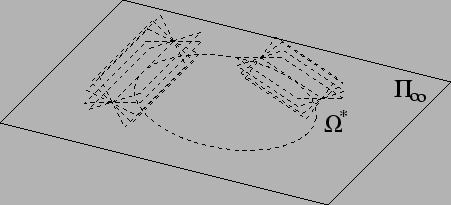Fig 5.2 在理想面上有一个圆锥线，仿射变换前后圆锥线轨迹可能会改变。

注意到在没有其他约束的情况下，此圆锥线轨迹已经变了。其实这一点也很容易理解，我们对仿射几何空间的坐标轴进行拉伸收缩，每一个轴进行的幅度是不同的，因此在理想面上的圆锥线自然会发生拉伸形变，比如说，可能会从圆形变成椭圆形。（事实上，圆锥曲线在投影变换下等价，这点也容易证明，暂且忽略）。
至于仿射变换的平行性不变性，这点非常容易证明，就留个读者证明吧。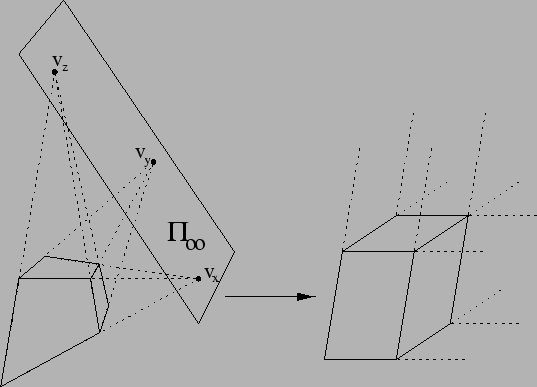Fig 5.3 这里阐述了描述一个正方体，在投影几何空间和仿射几何空间中的表示方式。

相似性变换
正如我们之前所说的，相似性变换是在欧几里德空间的每个坐标轴都拉伸收缩相同的幅度产生的，那么自然地，理想面上的圆锥曲线形状是不会改变的，至于这个圆锥曲线变得“多大多小”，这种尺度小大的是不影响不变性的。相似性变换的公式如：
\begin{aligned}\mathbf{T}_{M} &= \left[\begin{matrix}\sigma r_{11} & \sigma r_{12} & \sigma r_{13} & t_X \\\sigma r_{21} & \sigma r_{22} & \sigma r_{23} & t_Y \\\sigma r_{31} & \sigma r_{22} & \sigma r_{33} & t_Z \\0 & 0 & 0 & 1 \end{matrix}\right] \in \mathbb{R}^{4 \times 4} \\\left[\begin{matrix}X^{\prime} \\Y^{\prime} \\Z^{\prime} \\1\end{matrix}\right] &= \mathbf{T}_M\left[\begin{matrix}X \\Y \\Z \\1\end{matrix}\right]\end{aligned}\tag{5.7}
其中，注意到$\mathbf{T}_{M}$存在一些约束：
\begin{aligned} \mathbf{R}_{M} &= \left[ \begin{matrix} r_{11} & r_{12} & r_{13} \\ r_{21} & r_{22} & r_{23} \\ r_{31} & r_{32} & r_{33} \end{matrix} \right] \\ \mathbf{R}_M^{\mathrm{T}} \mathbf{R}_{M} &= \mathbf{R}_{M} \mathbf{R}_M^{\mathrm{T}} = \mathbf{I}_{3 \times 3} \\ \det(\mathbf{R}_M) &= 1 \end{aligned} \tag{5.8}
也就是说$\mathbf{T}_M$的子矩阵$\mathbf{R}_M$是一个正交矩阵，并且其行列式值为1（可以视为进行过归一化）。事实上，这里的$\mathbf{R}_M$可以视为是旋转矩阵，而$\mathbf{t}_M = (t_X, t_Y, t_Z)^{\mathrm{T}}$可以视为是将变换前的原点坐标挪到该处，是一个平移偏置向量。而$\sigma$表示的正是对整个客体的放缩尺寸大小。于是我们发现，整个$\mathbf{T}_M$的自由度为7，其中3个是朝向相关的自由度，3个是平移相关的，而1个是尺度大小相关的。
考虑到相似性变换前后的不变性，除了继承了仿射变换的不变性之外，还添加了两个重要的新的不变性：**相对距离不变 **和 角度不变。相对距离不变指的是变换前后每个线条的比例是一定的，线条之间的距离的比例也是不变的；角度不变就很好理解了，变换前后，线条之间的夹角不变。
对比仿射变换，相似性变换的理想面的圆锥线有个非常重要的性质，其变换前后形状不变。我们可以尝试对此进行证明。正如Fig 5.2所示，我们假设理想面$\Pi_{\infty}$ 上的二次曲锥面为$\Omega$， 但是二次曲锥面是一个立体图形，不容易可视化，我们经常用其对偶二次曲锥面为$\Omega^{*}$表示，其表现为一系列的平面。理想面上的二次曲锥面称之为绝对二次曲锥面（Absolute Quadric）。
二次曲锥面是在三维情况下的，在平面上的情况，二次曲锥面就变成了圆锥线$\omega_{\infty}$，其对偶形式为一系列的直线，表示为$\omega_{\infty}^*$。我们将理想面上的圆锥线称之为绝对圆锥线（Image of Absolute Conic， IAC）。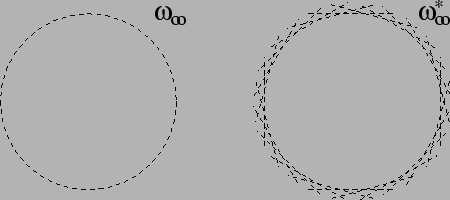Fig 5.4 理想面上的绝对圆锥线和其对偶形式

我们暂且只考虑三维情况下的$\Omega$，其最简单的形式就是一个球：
$\Omega : X^2+Y^2+Z^2 = 0 \tag{5.9}$
其矩阵形式为：
$\Omega = \left[ \begin{matrix} 1 & 0 & 0 & 0 \\ 0 & 1 & 0 & 0 \\ 0 & 0 & 1 & 0 \\ 0 & 0 & 0 & 0 \\ \end{matrix} \right] \tag{5.10}$
二次曲锥面的方程是$\mathbf{x}^{\mathrm{T}} \mathbf{\Omega} \mathbf{x} = 0$，经过相似性变换后，$\mathbf{x}^{\prime} = \mathbf{T}_M^{\mathrm{T}} \mathbf{x}$。于是变换后，新的二次曲锥面可以表示为：
$(\mathbf{T}_M^{\mathrm{T}} \mathbf{x})^{\mathrm{T}} \mathbf{\Omega} \mathbf{T}_M^{\mathrm{T}} \mathbf{x} = 0 \tag{5.11}$
$\mathbf{\Omega}^{\prime} = \mathbf{T}_{M} \mathbf{\Omega} \mathbf{T}_M^{\mathrm{T}} \tag{5.12}$
为了方便，我们将用分块矩阵的方式计算这个矩阵：
$\left[ \begin{matrix} \sigma \mathbf{R} & \mathbf{t}_M \\ 0_3^{\mathrm{T}} & 1 \end{matrix} \right] \left[ \begin{matrix} \mathbf{I}_{3 \times 3} & 0_3 \\ 0_3^{\mathrm{T}} & 0 \end{matrix} \right] \left[ \begin{matrix} \sigma \mathbf{R} & \mathbf{t}_M \\ 0_3^{\mathrm{T}} & 1 \end{matrix} \right]^{\mathrm{T}} \tag{5.13}$
于是有：
$\mathbf{\Omega}^{\prime} = \sigma^2 \mathbf{R} \mathbf{I}_{3 \times 3} \mathbf{R}^{\mathrm{T}} = \sigma^2 \mathbf{I}_{3 \times 3}\tag{5.14}$
因为$\sigma$是尺度因子，因此对形状没有影响，我们发现变换前后绝对圆锥线的形状不变。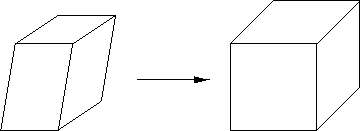Fig 5.5 左图是仿射变换，右图是相似性变换的结果。

欧几里德变换
欧几里德变换在相似性变换的基础上，只是把尺度因子$\sigma = 1$设置为了1， 其他不变，因此在继承了相似性变换的所有不变性特性的基础上，又增加了绝对长度不变性。我们有：
\begin{aligned}\mathbf{T}_{E} &= \left[\begin{matrix}r_{11} & r_{12} & r_{13} & t_X \\r_{21} & r_{22} & r_{23} & t_Y \\r_{31} & r_{22} & r_{33} & t_Z \\0 & 0 & 0 & 1 \end{matrix}\right] \in \mathbb{R}^{4 \times 4} \\\left[\begin{matrix}X^{\prime} \\Y^{\prime} \\Z^{\prime} \\1\end{matrix}\right] &= \mathbf{T}_E\left[\begin{matrix}X \\Y \\Z \\1\end{matrix}\right]\end{aligned}\tag{5.15}
因此其自由度就只剩下了3个方向自由度，3个平移自由度。

总结
洋洋洒洒地写了一大堆，现在总结下这四大变换的自由度和不变性：

投影变换，自由度15，不变性：交叉比，共线性，切线性。

仿射变换，自由度12，不变性：交叉比，共线性，切线性， 轴方向的相对距离不变，平行不变，理想面不变。

相似性变换，自由度7，不变性：交叉比，共线性，切线性， 轴方向的相对距离不变，平行不变，理想面不变， 相对距离不变，角度不变，绝对圆锥线不变。

欧几里德变换，自由度6，不变性：交叉比，共线性，切线性， 轴方向的相对距离不变，平行不变，理想面不变，相对距离不变，角度不变，绝对圆锥线不变，绝对距离不变。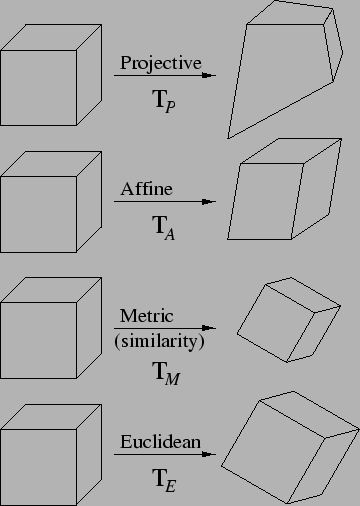PS: 本文引出绝对圆锥线的概念，是为了以后的立体视觉中的恢复重建任务和相机参数标定等任务进行铺垫。

Reference
. Hartley R, Zisserman A. Multiple View Geometry in Computer Vision[J]. Kybernetes, 2008, 30(9/10):1865 - 1872.
. https://www.cs.unc.edu/~marc/tutorial/node3.html
. https://blog.csdn.net/LoseInVain/article/details/102756630
. https://blog.csdn.net/LoseInVain/article/details/104515839
. https://blog.csdn.net/LoseInVain/article/details/102869987
. https://blog.csdn.net/LoseInVain/article/details/102883243
. https://blog.csdn.net/LoseInVain/article/details/102698703
. https://blog.csdn.net/LoseInVain/article/details/102739778
. https://blog.csdn.net/LoseInVain/article/details/102756630


展开全文立体几何 多视角几何 计算机视觉 计算机图形学
• 在本文首先介绍了引入齐次坐标系的必要性，随后介绍了在几何变换常见的投射变换和仿射变换，这俩种变换在计算机视觉问题中，包括在相机成像过程中都是很基础并且重要的内容。 如有谬误，请联系指正。转载请注明...

几何变换——关于透视变换和仿射变换以及齐次坐标系的讨论

2019/10/26 FesianXu

前言
在本文首先介绍了引入齐次坐标系的必要性，随后介绍了在几何变换中常见的投射变换和仿射变换，这俩种变换在计算机视觉问题中，包括在相机成像过程中都是很基础并且重要的内容。
如有谬误，请联系指正。转载请注明出处。
$\nabla$ 联系方式：
e-mail: FesianXu@gmail.com
QQ: 973926198
github: https://github.com/FesianXu
知乎专栏: 计算机视觉/计算机图形理论与应用
微信公众号：文章目录前言齐次坐标系的引入投影变换的背景坐标表达仿射变换和透视变换仿射变换尺度放缩(scale)平移(translate)切变(shear)旋转（rotate）可组合性透视变换广义投影变换Reference
齐次坐标系的引入
投影变换的背景
我们对于投影变换(projective transformation) 其实是一点的不陌生的，假设我们在看一张照片时，我们经常会发现本来应该是平行的线条，却变得不平行了，如Fig 1.1所示。更一般地说，在投影变换中，大部分的几何属性，比如长度，角度，比例，平行性等，都可能不能保留了，但是有一点我们是可以确保的，那就是 直线在变换前后始终还是直线。Fig 1.1 本来应该是平行的马路，在相机成像的时候，则变成了“不平行”，汇聚于无限远处的消失点(vanishing point)。Fig 1.2 现代的绘画作品很多采用了透视的方法增强了立体感，这是在仿照人类视觉的特点。

我们考虑到在欧式几何(Euclidean Geometry)中，其实有一些概念是有些“麻烦之处”的，比如说到平行这个概念，我们知道在欧几里德空间中，两个直线都会交于一点，当然除了平行线之外，这让平行线处于在一个很特殊的地位。如果我们熟悉编程我们就不难发现，当有个编程对象处于特殊地位时，我们就不得不单独考虑他，使得整个程序变得不能通用(general)起来，这个是个糟糕的事情。
当然，我们也可以耍滑头，说在欧几里德空间里，平行线相交于无限远的点处，这当然没问题。但是，“无限远”这种概念其实是为了方便定义出来的，实际上并不存在。不管怎么样，我们把平行线相交于的无限远的点，称之为 理想点(Ideal point)。
通过添加了这个理想点，我们把欧几里德空间(Euclidean Space)转变成了投影空间(Projective Space)，很简单吧，但是我们后面将会发现，我们的很多投影变换都只能在投影空间中进行，在没有定义出理想点的欧式空间，我们根本对这些变换无能为力。总而言之， 在投影空间中，所有的线最终都能够相交了。因为所有的理想点都有着相同的距离，所以在二维的投影空间中，所有的平行线其实都是交于由所有理想点组成的“理想直线”上的。同样的，在三维投影空间中，所有的平行面都交于一个“理想平面”。我们如果用符号$\mathbb{R}^{2}$表示二维的欧式空间，那么用$\mathbb{P}^{2}$表示二维的投影空间。
坐标表达
我们都知道一个在二维平面上的点可以用一组有序的二元对表示，如$(x,y)$。我们在此引入 齐次坐标(homogeneous coordinate) 的表达，我们将同样的二维点$(x,y)$表示成$(x,y,1)$，并且，其等价于$(kx, ky, k), k \neq 0$。 我们发现，基本上所有的齐次坐标表达$(x,y,c)$，都可以找到相对应的非齐次坐标表达方式$(x/c, y/c, 1)$，除了一个最为特殊的点$(x,y,0)$。这个点如果硬要用非齐次的方式去表示，那么只能表示为$(x/0, y/0, 1)$，我们发现，这个方式其实就是在齐次坐标系里面定义出了理想点这个概念，并且的，这个处于无限远处的理想点和普通点有着一致的表达方式，意味着可以用和普通点一样的处理方式去处理理想点这个概念了。
这个正是投影空间的精髓之处，在投影空间中，我们用齐次坐标去表示点，把空间中的点所有都看成是等价的，这样就不存在普通点与理想点的区别了，而且在这个空间中，所有的直线都会相交，因此也不存在平行性这个概念了。笔者在这里举个在投影空间处理点的变换的例子，假如现在在投影空间$\mathbb{P}^{n}$中定义了一个变换，我们首先用齐次坐标表示这个空间中的元素先，其是一个$(n+1)$维的向量，然后，我们定义这个变换，这个变换应该是一个矩阵，我们称之为单应性矩阵（homography），$\mathcal{H} \in \mathbb{R}^{(n+1) \times (n+1)}$，因此这个变换结果最后为:
$\mathbf{X}^{\prime} = \mathcal{H} \mathbf{X} \tag{1.1}$
通过这种方法，我们会发现我们同时可以考虑在欧式空间中的平行线和非平行线。这对处理投影变换非常的方便，因为投影变换是不保留平行性这个几何属性的，因此在变换的过程中，可能需要将平行线变换成非平行的。

仿射变换和透视变换
投影变换可以细分为 仿射变换(affine transform) 和 透视变换(perspective transform)，以及 广义的投影变换(general projective transform)。我们分别介绍下。
PS：注意，我们这里的点是二维点，也就是$\mathbf{x} = (u,v,1)^{\mathrm{T}} \in \mathbb{R}^3$，因此，单应性矩阵是$\mathcal{H} \in \mathbb{R}^{3 \times 3}$。如果延展到三维空间的点变换，那么点就是三维点，为$\mathbf{X} = (u,v,w,1)^{\mathrm{T}} \in \mathbb{R}^4$，单应性矩阵$\mathcal{H} \in \mathbb{R}^{4 \times 4}$。
仿射变换
仿射变换(affine transform)在变换前后，保留了元素的平行性，也就是说，在变换前是平行线的，在变换后同样也是平行线。仿射变换可以表示为一组线性变换，如(2.1)，同时，在齐次表达下，仿射变换通常可以用一个$2 \times 3$的矩阵表达（二维情况下），如(2.2)。
$\left[ \begin{matrix} x \\ y \end{matrix} \right] = \left[ \begin{matrix} a_{11} u + a_{12} v + a_{13} \\ a_{21} u + a_{22} v + a_{23} \end{matrix} \right] \tag{2.1}$
$\left[ \begin{matrix} x \\ y \\ 1 \end{matrix} \right] = \left[ \begin{matrix} a_{11} & a_{12} & a_{13} \\ a_{21} & a_{22} & a_{23} \\ 0 & 0 & 1 \end{matrix} \right] \left[ \begin{matrix} u \\ v \\ 1 \end{matrix} \right] \tag{2.2}$
通过对这六个元素的某些约束，比如令某些为0，为1等，我们将仿射变换又分成了以下若干种。
尺度放缩(scale)
尺度放缩(scale)的变换矩阵如：
$\mathcal{H} = \left[ \begin{matrix} a_{11} & 0 & 0\\ 0 & a_{22} & 0 \\ 0 & 0 & 1 \end{matrix} \right] \tag{2.3}$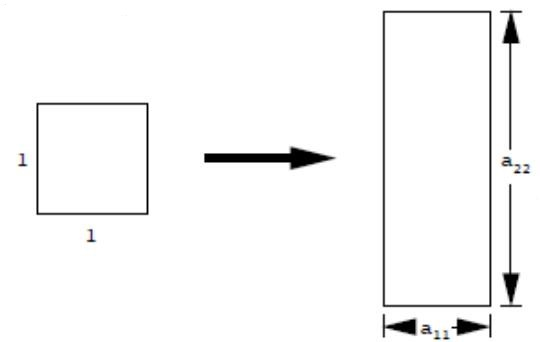Fig 2.1 尺度放缩示意图。

平移(translate)
平移(translate)的变换矩阵如：
$\mathcal{H} = \left[ \begin{matrix} 1 & 0 & a_{13}\\ 0 & 1 & a_{23} \\ 0 & 0 & 1 \end{matrix} \right] \tag{2.4}$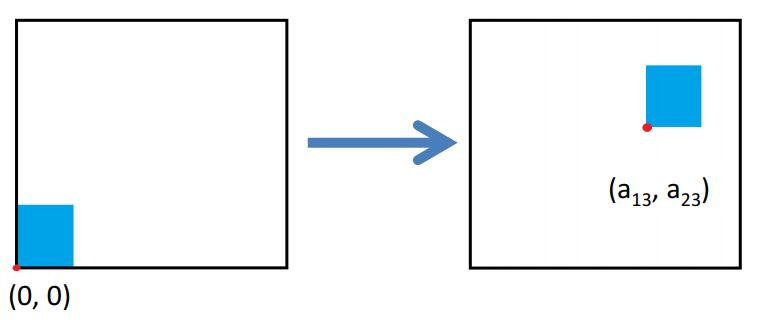Fig 2.2 平移示意图。

切变(shear)
切变(shear)的变换矩阵如：
$\mathcal{H} = \left[ \begin{matrix} 1 & a_{12} & 0\\ a_{21} & 1 & 0 \\ 0 & 0 & 1 \end{matrix} \right] \tag{2.5}$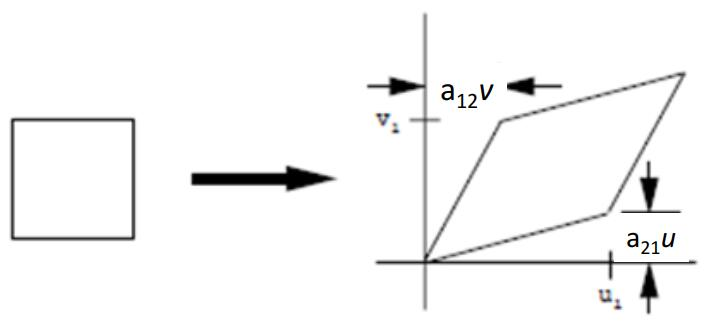Fig 2.3 切变示意图。

旋转（rotate）
旋转(rotate)的变换矩阵如：
$\mathcal{H} = \left[ \begin{matrix} \cos(\theta) & -\sin(\theta) & 0\\ \sin(\theta) & \cos(\theta) & 0 \\ 0 & 0 & 1 \end{matrix} \right] \tag{2.6}$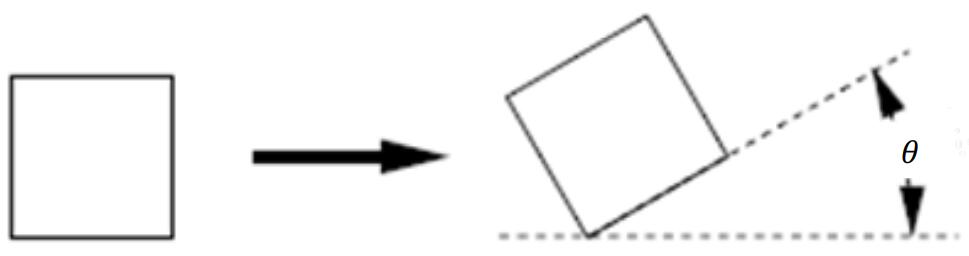Fig 2.4 旋转示意图。

可组合性
注意到仿射变换其是可以组合的，比如一个变换可以是先平移，后旋转，最后放缩，那么其变换矩阵为:
$\mathcal{H} = \mathcal{S}((\mathcal{R}(\mathcal{T}\mathbf{X}))) \tag{2.7}$
其中$\mathcal{S}$代表放缩变换，$\mathcal{R}$代表旋转变换，$\mathcal{T}$代表平移变换。
但是，我们也要注意到，一般来说其是不具有交换性的，比如先平移在旋转一般结果和先旋转后平移是不同的，即是：
$\mathcal{T}\mathcal{R} \neq \mathcal{R}\mathcal{T} \tag{2.8}$
其本质是矩阵乘法的不可交换性。
透视变换
透视变换(perspective transform)在变换前后不再保留所有元素的平行性了，但是变换前后，直线还是保持是直线，使用这种变换能提供3D的视觉效果。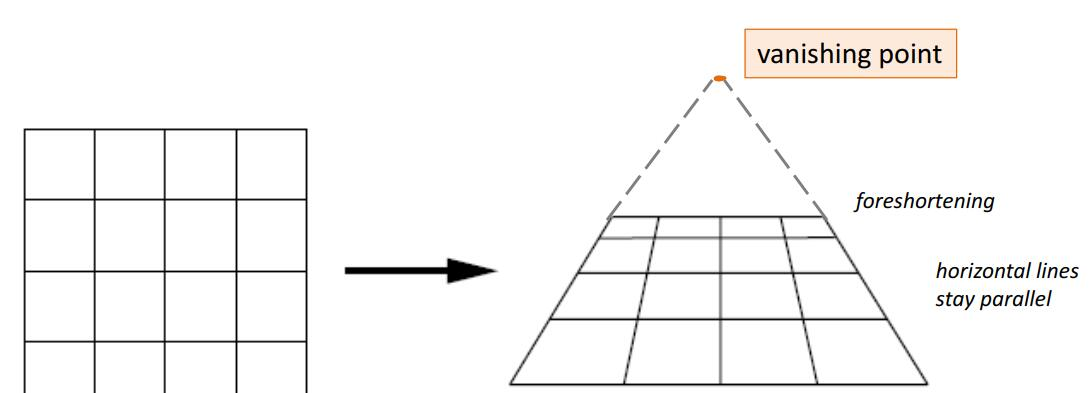Fig 3.1 透视变换，不能保证保留所有的平行性。其原先的平行线可能相交于理想点，也就是图中的消失点(vanishing point)。

我们同样用齐次坐标去表示这个变换，其变换矩阵的形式如:
$\left[ \begin{matrix} 1 & 0 & 0 \\ 0 & 1 & 0 \\ a_{31} & a_{32} & 1 \end{matrix} \right] \tag{3.1}$
观察变换过程(3.2)，我们发现本来在仿射变换中为1的$w$不再是1了。
$\left[ \begin{matrix} 1 & 0 & 0 \\ 0 & 1 & 0 \\ a_{31} & a_{32} & 1 \end{matrix} \right] \left[ \begin{matrix} u \\ v \\ 1 \end{matrix} \right] = \left[ \begin{matrix} u \\ v \\ a_{31} u + a_{32} v + 1 = w \end{matrix} \right] \tag{3.2}$
将其表示为非齐次的形式，我们有：
$\left[ \begin{matrix} u/w \\ v/w \\ 1 \end{matrix} \right]$
我们发现其是一个非线性变换，因此，透视变换其是一个非线性变换，处于无限远处的点可能会被移到有限处，处于有限点也可能会被移到无限点处，正是因为如此，平行性才不能被保留了。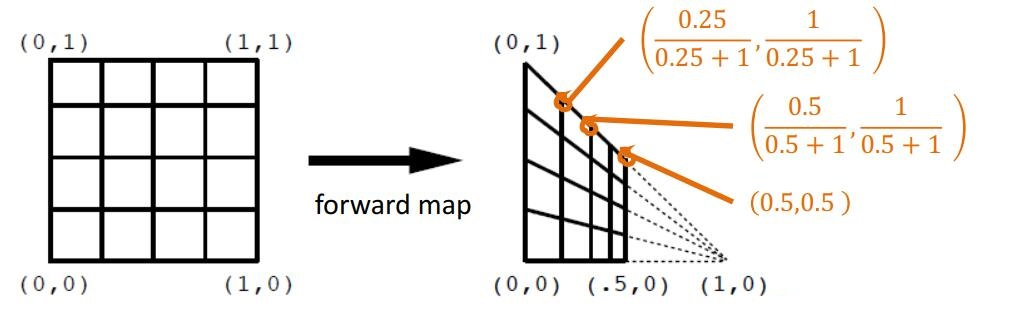Fig 3.2 透视变换的例子。

我们这里举个例子，假设有个透视变换矩阵:
$\mathcal{H} = \left[ \begin{matrix} 1 & 0 & 0 \\ 0 & 1 & 0 \\ 1 & 0 & 1 \end{matrix} \right]$
我们于是有转换过程:
$\mathcal{H} \left[ \begin{matrix} u \\ v \\ 1 \end{matrix} \right] = \left[ \begin{matrix} u \\ v \\ u+1 \end{matrix} \right] = \left[ \begin{matrix} \dfrac{u}{u+1} \\ \dfrac{v}{u+1} \\ 1 \end{matrix} \right] \tag{3.3}$
我们有:
\begin{aligned} \lim_{u \rightarrow \infty} \dfrac{u}{u+1} &= 1 \\ \lim_{u \rightarrow \infty} \dfrac{v}{u+1} &= 0 \end{aligned} \tag{3.4}
我们发现这个极限点正是其消失点的坐标。
广义投影变换
我们要注意到，透视变换其本质是广义的投影变换中的一种特殊情况，广义透视变换的变换矩阵如：
$\left[ \begin{matrix} a_{11} & a_{12} & a_{13} \\ a_{21} & a_{22} & a_{23} \\ a_{31} & a_{32} & a_{33} \end{matrix} \right] \tag{4.1}$
同样的，其也是一种非线性变换。在二维情况下我们有：
\begin{aligned} x &= \dfrac{a_{11} u + a_{12} v + a_{13}}{a_{31} u + a_{32} v + a_{33}} \\ y &= \dfrac{a_{21} u + a_{22} v + a_{23}}{a_{31} u + a_{32} v + a_{33}} \end{aligned}

Reference
.  Hartley R, Zisserman A. Multiple View Geometry in Computer Vision[J]. Kybernetes, 2008, 30(9/10):1865 - 1872.


展开全文仿射变换 透视变换 投影变换 计算机视觉
• 一、常见几何变换 1.图像剪裁 2.图像旋转 3. 图像平移 4.图像金字塔缩减和扩展 二、 几何坐标变换的描述 三、 将几何变换应用于图像 四、 将二维图像引至世界坐标(创建空间参照对象) 五、查找空间转换的输出范围 ...
几何变换

一、常见几何变换1.图像剪裁
2.图像旋转
3. 图像平移
4.图像金字塔缩减和扩展

二、 几何坐标变换的描述
三、 将几何变换应用于图像
四、 将二维图像引至世界坐标(创建空间参照对象)
五、查找空间转换的输出范围
六、根据输入空间限制查找输出空间限制
七、图像坐标系统
八、输出图像位置
九、控制输出网格
十、图像内插
十一、图像融合

一、常见几何变换

1.图像剪裁

J = imcrop(I,rect);

根据rect裁剪图像I,根据[xmin ymin width height]空间坐标指定裁剪矩形的大小和位置 。裁剪后的图像包含输入图像中所有由矩形完全或部分包围的像素。

2.图像旋转

B = imrotate(A,angle,method);

使用指定的插值方法method旋转图像A。

3. 图像平移

B = imtranslate(A,translation);

按照translation中指定的平移向量平移图像A。

4.图像金字塔缩减和扩展

B = impyramid(A,direction);

计算高斯金字塔减少或扩大一个级别。direction确定impyramid执行缩小还是扩展。

二、 几何坐标变换的描述

tform=maketform(transform_type,params,...);

三、 将几何变换应用于图像

B = imwarp(A,tform)

插值方式默认为线性内插

四、 将二维图像引至世界坐标(创建空间参照对象)

R = imref2d(imageSize)

要为图像指定世界（非默认空间）坐标系，可以使用 空间参照。imref2d可以让您在世界坐标系中定义2维图像的位置。您还可以使用这些对象来指定图像分辨率，包括非方形像素形状。（另一种定义世界坐标系的方法是使用图像对象XData和 YData属性 - 参见使用XData和YData属性定义世界坐标。）一个imref2d对象封装了锚定到二维图像的行和列的固有坐标与世界坐标系中相同行和列位置的空间位置之间的关系。

五、查找空间转换的输出范围

outbounds = findbounds(tform,inbounds);

估计对应于给定空间变换和一组输入边界的输出边界。tform是一个空间转换结构。inbounds是一个2 num_dims×矩阵，用于指定输出图像的下限和上限。outbounds是完全包含由输入边界表示的变换矩形的最小矩形区域的估计值。既然outbounds只是一个估计，它可能不会完全包含已变换的输入矩形。

六、根据输入空间限制查找输出空间限制

[xLimitsOut,yLimitsOut] = outputLimits(tform,xLimitsIn,yLimitsIn);

估计对应于一组输入空间限制的输出空间限制

七、图像坐标系统

MATLAB图像的坐标原点位于左上角，如图：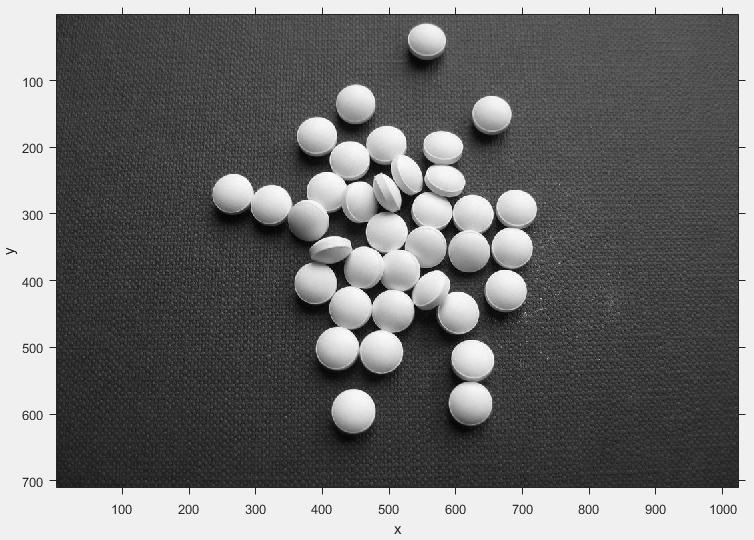这幅图像的大小为710*1024
左上角放大：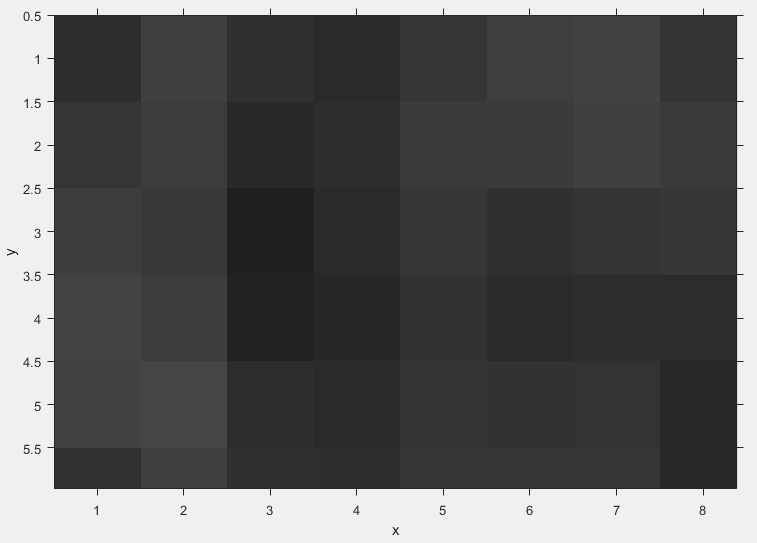在这里可以看到，像素都是方形的，而左上角的像素中心坐标为（1，1）。且所有像素中心都是整数值。

以下语句可改变默认空间坐标：

imshow(I,'XData',[-20,20],'YData',[-10,10]);

其中XData是二元素向量，第一个元素指定像素的第一列中心x的坐标，第二个元素指定像素最后一列中心x的坐标。 YData与其类似，但指定的是y的坐标。
效果图：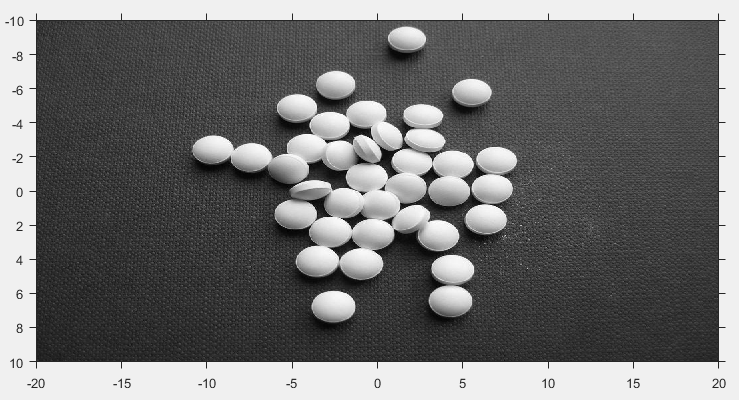左上角放大：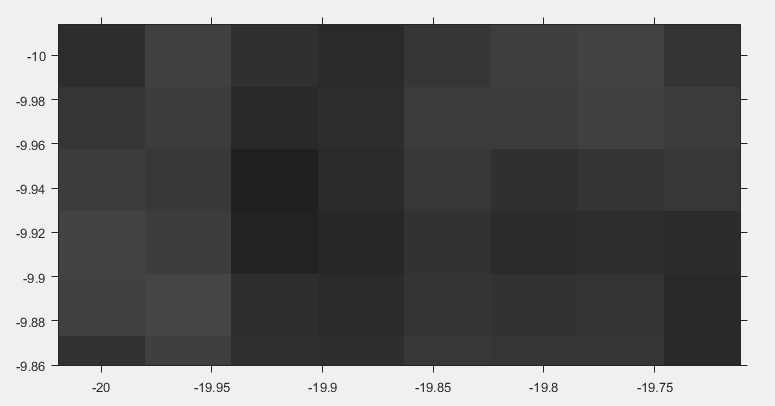可以看到，此时的像素不再是正方形，并且现在左上角坐标为（-10，-20）。像素的中心不再都是整数值。

八、输出图像位置

[g,xdata,ydata] = imtransform(f,tform);

经几何变换后得图像并未显示在输出空间的位置，使用此函数可以获取这个信息，即xdata,ydata。使用imshow显示图像时，第二第三个返回参数可以像XData,YData参数那样使用。如：

imshow(g,'XData',xdata,'YData',ydata);

通俗来说就是，若对图像进行了几何变换，输出的图像会默认包含变换后的图像，而使用这两个返回的参数则能够在原本输出空间显示图像。

九、控制输出网格

要达到同一效果，可以在输出空间用imtransform控制输出网格。即定制其XData与YData这两个输入参数。

十、图像内插

1.最近邻
2.双线性
3.双三次

十一、图像融合

1.直接平均融合法
2.加权平均法
3.中值滤波法
4.多分辨率处理法
展开全文MATLAB
• ## OpenGL几何变换

千次阅读 2015-09-29 21:44:09
OpenGL几何变换  OpenGL中指定了顶点之后，但在屏幕上显示它们之前，会发生三种类型的变换：视图变换、模型变换和投影变换。OpenGL中一些变换术语如下表： 变换 用途 视图(Viewing) 指定观察者或照相机的位置 ...opengl
• 几何变换有五种常见类型：剪切变换、平移变换、缩放变换、旋转变换和投影变换。 它们如图4.1所示。 在该图中，原始图像显示在(A)中，而变换后的图像显示在(B)到(F)中。 几何变换可以表示为原始像素位置和新像素位置...matlab 数字图像处理 maketform
• 图像的几何变换 二、实验目的 1.熟悉MATLAB软件的使用。 2.掌握图像几何变换的原理及数学运算。 3.于MATLAB环境下编程实现对图片不同的几何变换。 三、实验内容 1.将图像绕图像中心顺时针旋转30度，旋转之后的图像...MATLAB 数字图像处理
• 图像的几何变换一.简介二.算法理论介绍1.公式2.坐标系变换3.向前映射法和向后映射法向前映射法向后映射法4.插值三.基于OpenCV的python实现及效果展示平移旋转仿射变换透视变换 一.简介 几何空间变换是图像处理中的最...计算机视觉 python opencv
• 几何变换，又称空间变换，是图形处理的一个方面，是各种图形处理算法的基础。它将一幅图像中的坐标位置映射到另一幅图像中的新坐标位置，其实质是改变像素的空间位置，估算新空间位置上的像素值。 几何变换不改变...缩放
• 图像的几何变换     图像的几何变换又称为图像空间变换，它将一幅图像中的坐标位置映射到另一幅图像中的新生位置。几何变换不改变图像的像素值，只是在图像平面上进行像素的重新安排。  &...
• 在机器视觉系统中，镜头是重要成像部件之一，而基于小孔成像原理的工业镜头往往会产生透视畸变现象，如何校正畸变是进行图像分析的前提 ，这其中就会用到投影变换，也是几何变换的一种。除此之外，图像处理中常用到...
• 几何变换的形式1.1旋转变换2.变换中心2.1坐标系变换3.映射与插值 1.几何变换的形式 几何变换的一般形式如下： 式中的T即为变换矩阵，(v,w)是原坐标，(x,y)是变换后的坐标，不同的变换对应不同的矩阵，，一些常见的...
• 6.1 三维图形几何变换三维物体的几何变换是在二维方法基础上增加了对 z 坐标的考虑得到的。有关二维图形几何变换的讨论，基本上都适合三维空间。从应用角度来看，三维空间几何变化直接与显示和造型有关，因此更为...计算机图形学
• 【实验目的】 了解几何变换的基本概念。 了解平移、伸缩、对称、旋转等变换。 学习掌握MATLAB软件有关的命令。 【实验内容】 将函数的图形向右平移3个单位。 【实验准备】 ...2．几种常见几何变换 常见的...
• 常见几何变换有： 镜像，平移，旋转，缩放，仿射等变换。 本节介绍图像镜像翻转变换，作为知识储备。 代码实现 import numpy as np import cv2 as cv from matplotlib import pyplot...python openCV 数字图像处理
• 本代码为MATLAB实现图像的几何变换，包含图像的水平镜像、垂直错切、旋转和加倍，内附有图片经过代码处理后的结果
• 几何变换 使用 Canvas 来做常见的二维变换 注意的点 Canvastranslatefloat dx float dy 平移 Canvasrotatefloat degrees float px float py 旋转 Canvasscalefloat sx float sy float px float py 放缩 skewfloat sandroid canvas matrix
• 常见几何变换有平移、镜像、旋转、缩放、仿射等。图像的几何变换常作为图像处理的预步骤，应用十分广泛。下面将介绍以上几种常见变换的原理及程序实现。 1.坐标映射 图像的几何变换改变了像素的空间位置，建立一...
• 在传统CV领域，由于某些拍摄角度的问题，我们需要对图像进行矫正处理，而几何变换正是这个处理过程的基础，因此了解和学习几何变换也是有必要的。 这次我们带着几个问题进行，以旋转为例： 1：变换的形式（公式）是...python opencv 计算机视觉 人工智能 c++
• 目录 1 简介 2 常见仿射变换 3 算法理论介绍 3.1 变换形式 ...在传统CV领域，由于某些拍摄角度的问题，我们需要对图像进行矫正处理，而几何变换正是这个处理过程的基础，因此了解和学习几何变换计算机视觉
• 什么是几何变换（Transform）在图形学中，主要有三种几何变换，分别是平移（Translate），旋转（Rotation）和缩放（Scaling）。在D2D中，这三种变换都有实现，而且还有一种不太常见的变换，倾斜（Skewing）。 ...
• 图像的几何变换是指在不改变图像像素的前提下对图像像素进行空间几何变换常见的变换有距离变换，坐标映射，平移，镜像，旋转，缩放和仿射变换等等。 也就是说，图像的几何变换就是建立一种源图像像素与变换后的...opencv 图像
• 文章目录图像的几何变换1 图像平移1.1 图像平移的变换公式1.2 MATLAB实现2 图像镜像2.1 图像镜像的变换公式2.2 MATLAB编程实现3 图像转置3.1 图像转置的变换公式3.2 MATLAB编程实现4 图像缩放4.1 图像缩放的变换公式...
• 【图像处理】图像几何变换基础（非弹性变换下如何计算旋转矩阵）图像处理 图像配准
• 图像几何变换介绍     图像的几何空间变换是图像处理中的最基础的算法，是指对原始图像按需要改变其大小、形状和位置的变化，原始图像与目标函数之间的坐标变换函数为线性函数。二维图像的基本...
• 图像的几何变换都可以用X=imtransform（I, TFORM , method）; 函数完成 I：要进行几何变换的图片 TFORM ：选择变换的类型 method：允许为imtransform函数选择的插值方法，以下三种可填： ‘nearest’ 最近邻插值 ...matlab
• % 调用方法：OutImage = ImageShiftFun(InputImage,deltaX,deltaY) % 日期：2019.11.26 % 作者：Leetion [iLimit,jLimit] = size(InputImage); switch method case 1 OutImage = zeros(iLimit, jLimit); ...数字图像处理 Matlab
• 计算机视觉基础-图像处理-几何变换（平移和旋转）一、简介二、算法理论介绍2.1变换形式2.2坐标系变换2.3映射方法——反向映射2.4插值算法三、基于OpenCV的代码实现 一、简介 几何变换的原理大多都是相似，只是变换...
• OpenCV2:图像的几何变换,平移、镜像、缩放、旋转(1) 图像的几何变换是在不改变图像内容的前提下对图像像素的进行空间几何变换，主要包括了图像的平移变换、镜像变换、缩放和旋转等。本文首先介绍了图像几何...图像...